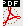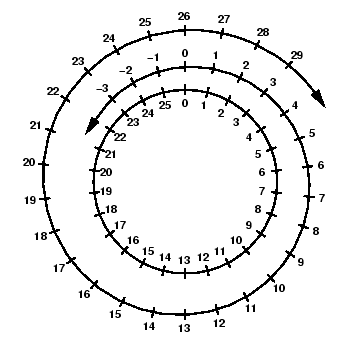2 I'm a SubstituteV'ir Tbg n FrpergThis document as PDF4 Many Caesars: the

# 3 The Caesar Cipher and Modular Arithmetic

In order to better understand substitution ciphers, and make something better out of them, we do what is very common in mathematics: we make the problem easier, and don't worry (at first) that it seems like a step backwards.

So, instead of considering all possible substitutions, we just think about some simple ones: those where we leave the alphabet in order, and just shift by a few letters. Julius Caesar is reported to have used such a cipher with a shift of 3 for his military communications.

The correspondence between plaintext and ciphertext is as follows:

abcde fghij klmno pqrst uvwxy z
XYZAB CDEFG HIJKL MNOPQ RSTUV W

If we encrypt veni vidi vici using the Caesar cipher, we get SBKF SFGF SFZF. To decrypt, we just shift back by 3 letters.

So where's the math? Let's see if we can find it.

First, we assign a number to each letter of the plaintext alphabet, beginning with 0, so we that have the correspondence below.
 a b c d e f g h i j k l m n o p q r s t u v w x y z 0 1 2 3 4 5 6 7 8 9 10 11 12 13 14 15 16 17 18 19 20 21 22 23 24 25

Now, we can view our enciphering as taking the number corresponding to the letter, adding 3 to it, and then writing down the letter that corresponds to the sum. If the result is 26 or larger, we subtract 26 so that if falls back in the desired range.

This type of arithmetic is called addition modulo 26 or just adding mod 26. The number 26 is called the base. You are already quite familiar with another type of modular arithmetic, namely working mod 12. When we answer a question like What time will it be 4 hours after 10 o'clock?'', we are adding mod 12. Another way to view modular arithmetic is that we do regular'' arithmetic, divide the answer by the base, and then only keep the remainder.6 We sometimes also work modulo 7 when we make statements like My birthday is on a Friday this year, so next year it will be on Saturday''- here we are using the fact that 365 = 7 x 52 + 1, so. Waiting a year advances the day of the week by one (except in a leap year).

.5Returning to our encryption problem, we see that applying the Caesar cipher corresponds just to adding 3 (mod 26). To decipher, we can either subtract 3 modulo 26 (remembering to add 26 to the answer if it turns out negative), or we can add 23, which is 26-3. These give exactly the same answer after reducing modulo 26, in the same way that a clock will read the same if you move the hands ahead by 3 hours or back 9 hours.

A useful mental model for modular addition is a number circle''. Take the familiar number line and wrap it around a circle so that the base (26) falls on zero. Then adding corresponds to a clockwise rotation, and subtraction to counterclockwise rotation.

Naturally, we don't have to shift by 3 as Julius Caesar did (apparently Augustus Caesar preferred to shift by 1). We have 25 possible ciphers like the Caesar cipher, which are called shift ciphers, or sometimes the general shift cipher is called a Caesar cipher''.7If someone says the Caesar cipher, they almost certainly mean a shift cipher with a shift of 3.

Notice that shift ciphers are very easy to break, since you only have to guess one letter and then you know everything. If you haven't already discovered it, the title of this note is encrypted with a shift cipher, leaving the spaces and punctuation in. So that you don't have to look back, the (encrypted) title is

V'IR TBG N FRPERG

#### Footnotes

... remainder.6
Modular arithmetic works for multiplication as well as for addition and subtraction. One has to be careful about what division means, however. We'll cover this further in section 6.
... cipher''.7
One shift cipher commonly used in internet postings or emails that may tell the ending of a movie or the answer to a puzzle is called rot13'' (for rotation by 13). This is just a Caesar-like cipher with a shift of 13 (leaving all the non-alphabetic text alone). This is popular because applying the same shift again decodes the text.2 I'm a SubstituteV'ir Tbg n FrpergThis document as PDF4 Many Caesars: the
Scott Sutherland
2005-10-26